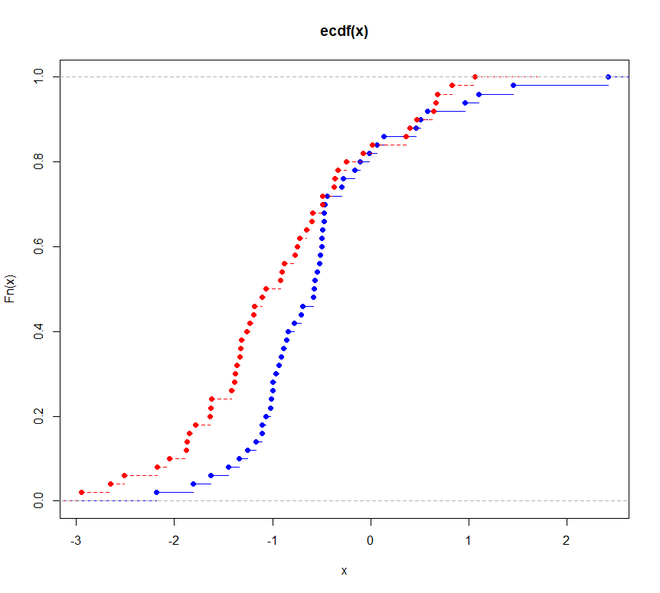# Kolmogorov-Smirnov Test in R Programming

The Kolmogorov-Smirnov Test is a type of non-parametric test of the equality of discontinuous and continuous of a 1D probability distribution that is used to compare the sample with the reference probability test (known as one-sample K-S Test) or among two samples (known as two-sample K-S test). A K-S Test quantifies a distance between the cumulative distribution function of the given reference distribution and the empirical distributions of given two samples, or between the empirical distribution of given two samples. In a one-sample K-S test, the distribution that is considered under a null hypothesis can be purely discrete or continuous or mixed. In the two-sample K-S test, the distribution considered under the null hypothesis is generally continuous distribution but it is unrestricted otherwise. The Kolmogorov-Smirnov test can be done very easily in R Programming.

#### Kolmogorov-Smirnov Test Formula

The formula for the Kolmogorov-Smirnov test can be given as:where,

supx : the supremum of the set of distances

Fn(x) : the empirical distribution function for n id observations Xi

The empirical distribution function is a distribution function that is associated with the empirical measures of the chosen sample. Being a step function, this cumulative distribution jumps up by a 1/n step at each and every n data points.

#### Implementation in R

The K-S test can be performed using the ks.test() function in R.

Syntax:

ks.text(x, y, …, alternative = c(“two.sided”, “less”, “greater”), exact= NULL, tol= 1e-8,
simulate.p.value = FALSE, B=2000)

Parameters:

x: numeric vector of data values
y: numeric vector of data values or a character string which is used to name a cummulative distribution function.
…: the parameters which are defined by the y value

alternative: used to indicate the alternate hypothesis.
exact: usually NULL or it indicates a logic that an exact p-value should be computed.

tol: an upper bound used for rounding off errors in the data values.
simulate.p.value: a logic that checks whether to use Monte Carlo method to compute the p-value.
B: an integer value that indicates the number of replicates to be created while using the Monte Carlo method.

Let us understand how to execute a K-S Test step by step using an example of a two-sample K-S test.

• Step 1: At first install the required packages. For performing the K-S test we need to install the “dgof” package using the install.packages() function from the R console.
```install.packages("dgof")
```
• Step 2: After a successful installation of the package, load the required package in our R Script. for that purpose, use the library() function as follows:

## R

 `# loading the required package ` `library``(``"dgof"``)`

• Step 3: Use the rnorm() function and the runif() function to generate to samples say x and y. The rnorm() function is used to generate random variates while the runif() function is used to generate random deviates.

## R

 `# loading the required package ` `library``(dgof)  ` ` `  `# generating random variate ` `# sample 1 ` `x <- ``rnorm``(50) ` ` `  `# generating random deviates ` `# sample 2 ` `y <- ``runif``(30)`

• Step 4: Now perform the K-S test on these two samples. For that purpose, use the ks.test() of the dgof package.

## R

 `# loading the required package ` `library``(dgof)  ` ` `  `# generating random variate ` `# sample 1 ` `x <- ``rnorm``(50) ` ` `  `# generating random deviates ` `# sample 2 ` `y <- ``runif``(30) ` ` `  `# performing the K-S Test ` `# Do x and y come from  ` `# the same distribution? ` `ks.test``(x, y)`

Output:

```    Two-sample Kolmogorov-Smirnov test

data:  x and y
D = 0.84, p-value = 5.151e-14
alternative hypothesis: two-sided
```

#### Visualization of the Kolmogorov- Smirnov Test in R

Being quite sensitive to the difference of shape and location of the empirical cumulative distribution of the chosen two samples, the two-sample K-S test is efficient, and one of the most general and useful non-parametric test. Hence we will see how the graph represents the difference between the two samples.

Example:

Here we are generating both the samples using the rnorm() functions and then plot them.

## R

 `# loading the required package ` `library``(dgof)  ` ` `  `# sample 1 ` `# generating a random variate ` `x <- ``rnorm``(50) ` ` `  `# sample 2 ` `# generating a random variate ` `x2 <- ``rnorm``(50, -1) ` ` `  `# plotting the result ` `# visualization ` `plot``(``ecdf``(x),  ` `     ``xlim = ``range``(``c``(x, x2)),  ` `     ``col = ``"blue"``) ` `plot``(``ecdf``(x2),  ` `     ``add = ``TRUE``,  ` `     ``lty = ``"dashed"``, ` `     ``col = ``"red"``) ` ` `  `# performing the K-S  ` `# Test on x and x2 ` `ks.test``(x, x2, alternative = ``"l"``)`

Output:

```    Two-sample Kolmogorov-Smirnov test

data:  x and x2
D^- = 0.34, p-value = 0.003089
alternative hypothesis: the CDF of x lies below that of y
```My Personal Notes arrow_drop_upCheck out this Author's contributed articles.

If you like GeeksforGeeks and would like to contribute, you can also write an article using contribute.geeksforgeeks.org or mail your article to contribute@geeksforgeeks.org. See your article appearing on the GeeksforGeeks main page and help other Geeks.

Please Improve this article if you find anything incorrect by clicking on the "Improve Article" button below.

Article Tags :
Practice Tags :

Be the First to upvote.

Please write to us at contribute@geeksforgeeks.org to report any issue with the above content.